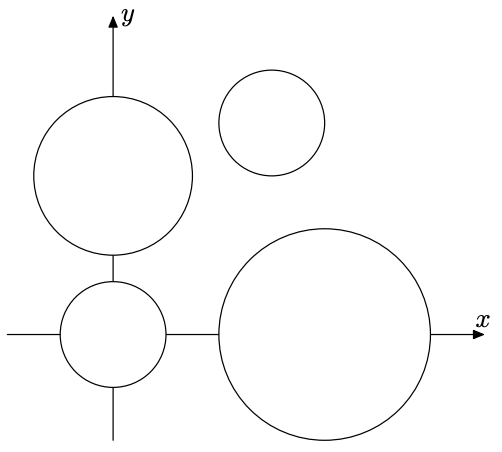시간 제한 메모리 제한 제출 정답 맞은 사람 정답 비율
8 초 (추가 시간 없음) 512 MB 0 0 0 0.000%

## 문제

You are given N non-overlapping circles in xy-plane. The radius of each circle varies, but the radius of the largest circle is not double longer than that of the smallest.Figure 1: The Sample Input

The distance between two circles C1 and C2 is given by the usual formula

$$\sqrt{(x_1 - x_2)^2 + (y_1 - y_2)^2} - r_1 - r_2$$

where (xiyi) is the coordinates of the center of the circle Ci, and ri is the radius of Ci, for i = 1, 2.

Your task is to write a program that finds the closest pair of circles and print their distance.

## 입력

The input consists of a series of test cases, followed by a single line only containing a single zero, which indicates the end of input.

Each test case begins with a line containing an integer N (2 ≤ N ≤ 100000), which indicates the number of circles in the test case. N lines describing the circles follow. Each of the N lines has three decimal numbers RX, and Y. R represents the radius of the circle. X and Y represent the x- and y-coordinates of the center of the circle, respectively.

## 출력

For each test case, print the distance between the closest circles. You may print any number of digits after the decimal point, but the error must not exceed 0.00001.

## 예제 입력 1

4
1.0 0.0 0.0
1.5 0.0 3.0
2.0 4.0 0.0
1.0 3.0 4.0
0


## 예제 출력 1

0.5